# Sinc function

(diff) ← Older revision | Latest revision (diff) | Newer revision → (diff)
Jump to: navigation, search
This article is about a particular function from a subset of the real numbers to the real numbers. Information about the function, including its domain, range, and key data relating to graphing, differentiation, and integration, is presented in the article.
View a complete list of particular functions on this wiki
For functions involving angles (trigonometric functions, inverse trigonometric functions, etc.) we follow the convention that all angles are measured in radians. Thus, for instance, the angle of$90\,^\circ$ is measured as$\pi/2$.

## Definition

This function, denoted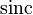$\operatorname{sinc}$, is defined as follows:$\operatorname{sinc} x := \left\lbrace \begin{array}{rl} 1, & x = 0 \\ \frac{\sin x}{x} & x \ne 0\end{array}\right.$

## Key data

Item Value
default domain all real numbers, i.e., all of$\R$.
range the closed interval$[\alpha,1]$ where$\alpha$ is approximately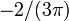$-2/(3\pi)$.
period none; the function is not periodic
horizontal asymptotes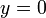$y = 0$, i.e., the$x$-axis. This is because$\lim_{x \to \pm \infty} \frac{\sin x}{x} = 0$, which in turn can be deduced from the fact that the numerator is bounded while the magnitude of the denominator approaches$\infty$.
local maximum values and points of attainment The local maxima occur at points$x$ satisfying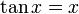$\tan x = x$ and$x \in [2n\pi,(2n + 1)\pi]$ or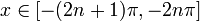$x \in [-(2n + 1)\pi,-2n\pi]$ for$n$ a positive integer.
There is an anomalous local maximum at$x = 0$ with value 1. Apart from that, the other local maxima occur at points of the form$\pm(2n\pi + \alpha_n)$ where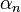$\alpha_n$ is fairly close to$\pi/2$ for all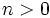$n > 0$. The local maximum value at this point is slightly more than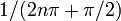$1/(2n\pi + \pi/2)$.
local minimum values and points of attainment The local minima occur at points$x$ satisfying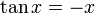$\tan x = -x$ and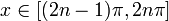$x \in [(2n - 1)\pi,2n\pi]$ or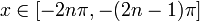$x \in [-2n\pi,-(2n - 1)\pi]$ for$n$ a positive integer.
The local minima occur at points of the form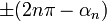$\pm(2n\pi - \alpha_n)$ where$\alpha_n$ is fairly close to$\pi/2$ for all$n > 0$. The local minimum value at this point is slightly less than$-1/(2n\pi - \pi/2)$.
points of inflection Fill this in later
important symmetries The function is an even function, i.e., the graph has symmetry about the$y$-axis.
derivative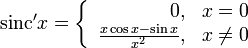$\operatorname{sinc}'x = \left\lbrace \begin{array}{rl} 0, & x = 0 \\ \frac{x \cos x - \sin x}{x^2}, & x \ne 0\\\end{array}\right.$
antiderivative the sine integral (this is defined as the antiderivative of the sinc function that takes the value 0 at 0)
power series and Taylor series The power series about 0 (which is also the Taylor series) is$\sum_{k=0}^\infty \frac{(-1)^kx^{2k}}{(2k + 1)!} = 1 - \frac{x^2}{3!} + \frac{x^4}{5!} - \dots$
The power series converges globally to the function.

## Graph

Below is a graph of the function for the domain restricted to$[-3\pi,3\pi]$:

The picture is a little unclear, so we consider an alternative depiction of the graph where the$x$-axis and$y$-axis are scaled differently to make it clearer:

## Differentiation

### First derivative

Computation at$x = 0$:

WARNING ON ERRONEOUS DIFFERENTIATION APPROACH: Suppose a function is given a separate definition at an isolated point from the definition at points surrounding it (on its immediate left or immediate right). To compute the derivative, it is not correct to simply differentiate the definition at the point and arrive at the derivative. Rather, we need to compute the derivative from first principles as a limit of a difference quotient, where the function value at the point is taken as the specified value and the function value at nearby points is given by the expression used to define the function around the point.

Here, we need to compute the derivative using first principles, as a limit of a difference quotient:$\operatorname{sinc}' 0 := \lim_{x \to 0} \frac{\operatorname{sinc} x - \operatorname{sinc} 0}{x - 0} = \lim_{x \to 0} \frac{(\sin x)/x - 1}{x} = \lim_{x \to 0} \frac{\sin x - x}{x^2}$

This limit can be computed in many ways. For instance, it can be computed using the L'Hopital rule: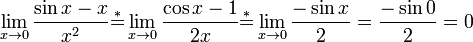$\lim_{x \to 0} \frac{\sin x - x}{x^2} \stackrel{*}{=} \lim_{x \to 0} \frac{\cos x - 1}{2x} \stackrel{*}{=} \lim_{x \to 0} \frac{-\sin x}{2} = \frac{-\sin 0}{2} = 0$

DERIVATIVE OF EVEN FUNCTION AT ZERO: For an even function, if the function is differentiable at zero, then the derivative at zero is zero. However, it is possible for an even function to not be differentiable at zero, so we cannot directly conclude merely from the function being even that its derivative at zero is zero.

Computation at$x \ne 0$: At any such point, we know that the function looks like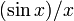$(\sin x)/x$ in an open interval about the point, so we can use the quotient rule for differentiation, which states that: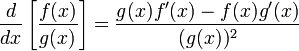$\frac{d}{dx}\left[\frac{f(x)}{g(x)}\right] = \frac{g(x)f'(x) - f(x)g'(x)}{(g(x))^2}$

In this case,$f(x) = \sin x$ and$g(x) = x$, so we get:$\frac{d}{dx}(\operatorname{sinc}\ x) = \frac{x \cos x - \sin x}{x^2}$

Combining the two computations, we get: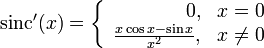$\operatorname{sinc}'(x) = \left\lbrace \begin{array}{rl} 0, & x = 0 \\ \frac{x \cos x - \sin x}{x^2}, & x \ne 0 \\\end{array}\right.$

### Second derivative

Computation at$x = 0$:

WARNING ON ERRONEOUS DIFFERENTIATION APPROACH: Suppose a function is given a separate definition at an isolated point from the definition at points surrounding it (on its immediate left or immediate right). To compute the derivative, it is not correct to simply differentiate the definition at the point and arrive at the derivative. Rather, we need to compute the derivative from first principles as a limit of a difference quotient, where the function value at the point is taken as the specified value and the function value at nearby points is given by the expression used to define the function around the point.

Here, we need to compute the derivative using first principles, as a limit of a difference quotient: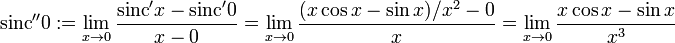$\operatorname{sinc}'' 0 := \lim_{x \to 0} \frac{\operatorname{sinc}' x - \operatorname{sinc}' 0}{x - 0} = \lim_{x \to 0} \frac{(x \cos x - \sin x)/x^2 - 0}{x} = \lim_{x \to 0} \frac{x \cos x - \sin x}{x^3}$

The latter limit can be computed in many ways. One way is to use the L'Hopital rule:$\lim_{x \to 0} \frac{x \cos x - \sin x}{x^3} \stackrel{*}{=} \lim_{x \to 0} \frac{-x \sin x + \cos x - \cos x}{3x^2} = \lim_{x \to 0} \frac{-\sin x}{3x} \stackrel{*}{=} \lim_{x \to 0} \frac{-\cos x}{3} = \frac{-1}{3}$

Thus,$\operatorname{sinc}'' 0 = -1/3$.

The computation at other points can be done using the usual method for computing derivatives, i.e., with the quotient rule for differentiation combined with other rules. Overall, we get: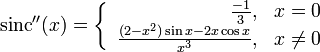$\operatorname{sinc}''(x) = \left\lbrace \begin{array}{rl} \frac{-1}{3}, & x = 0 \\ \frac{(2 - x^2)\sin x - 2x\cos x}{x^3}, & x \ne 0 \\\end{array}\right.$

### Higher derivatives at zero

The limit procedure used for the first and second derivatives can be extended to the computation of higher derivatives at zero. However, this process is tedious. An alternative approach is to use the power series expansion:$\operatorname{sinc} x = 1 - \frac{x^2}{3!} + \frac{x^4}{5!} - \dots = \sum_{k=0}^\infty \frac{(-1)^kx^{2k}}{(2k + 1)!}$

Since this is a power series, it is also a Taylor series. Thus, the coefficient of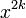$x^{2k}$ is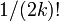$1/(2k)!$ times$\operatorname{sinc}^{(2k)}(0)$. We thus get:$\frac{1}{(2k + 1)!} = \frac{(-1)^k \operatorname{sinc}^{(2k)}(0)}{(2k)!}$

Rearranging and simplifying, we get:$\operatorname{sinc}^{(2k)}(0) = \frac{(-1)^k}{2k + 1}$

This gives an explicit expression for the even order derivatives. The odd order derivatives (first derivative, third derivative, etc.) are zero because the function is an even function.

The first few derivative values at zero (starting from the zeroth) are:$n$ 0 1 2 3 4 5 6 7 8 9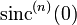$\operatorname{sinc}^{(n)}(0)$ 1 0 -1/3 0 1/5 0 -1/7 0 1/9 0

## Integration

### First antiderivative

These is no antiderivative for this among elementary functions. However, we can create a new function that serves as the antiderivative:$\operatorname{Si}(x) := \int_0^x \operatorname{sinc} t \, dt$

The function$\operatorname{Si}$ is termed the sine integral.

### Integration of transformed versions of function

We have:$\int_0^x \operatorname{sinc}(mt) \, dt = \frac{1}{m} \operatorname{Si}(mx)$

In indefinite integral form:$\int \operatorname{sinc}(mx + \varphi) \, dx = \frac{1}{m} \operatorname{Si}(mx + \varphi)$

### Improper definite integrals

The following fact is true, but not easy to prove:$\int_0^\infty \operatorname{sinc} x \, dx = \frac{\pi}{2}$

Because the function is an even function, this is equivalent to the following:$\int_{-\infty}^0 \operatorname{sinc} x \, dx = \frac{\pi}{2}$

and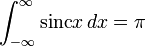$\int_{-\infty}^\infty \operatorname{sinc} x \, dx = \pi$

### Second antiderivative

The second antiderivative is: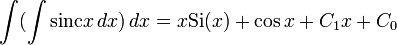$\int (\int \operatorname{sinc} x \, dx) \, dx = x \operatorname{Si}(x) + \cos x + C_1x + C_0$

To obtain this, we use integration by parts to integrate the function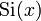$\operatorname{Si}(x)$.

### Higher antiderivatives

Higher antiderivatives of the sinc function can be computed in the same manner using integration by parts. Up to the arbitrary polynomial additive, the antiderivative is expressible as a polynomial linear combination of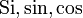$\operatorname{Si}, \sin, \cos$.

## Taylor series and power series

### Computation of power series

We use the power series for the sine function (see sine function#Computation of power series):$\! \sin x := x - \frac{x^3}{3!} + \frac{x^5}{5!} - \frac{x^7}{7!} + \dots = \sum_{k=0}^\infty \frac{(-1)^kx^{2k+1}}{(2k + 1)!}$

Dividing both sides by$x$ (valid when$x \ne 0$), we get:$\! \frac{\sin x}{x} = 1 - \frac{x^2}{3!} + \frac{x^4}{5!} - \frac{x^6}{7!} + \dots = \sum_{k=0}^\infty \frac{(-1)^kx^{2k}}{(2k+1)!}$

We note that the power series also works at$x = 0$ (because$\operatorname{sinc} \ 0 = 1$), hence it works globally, and is the power series for the sinc function.